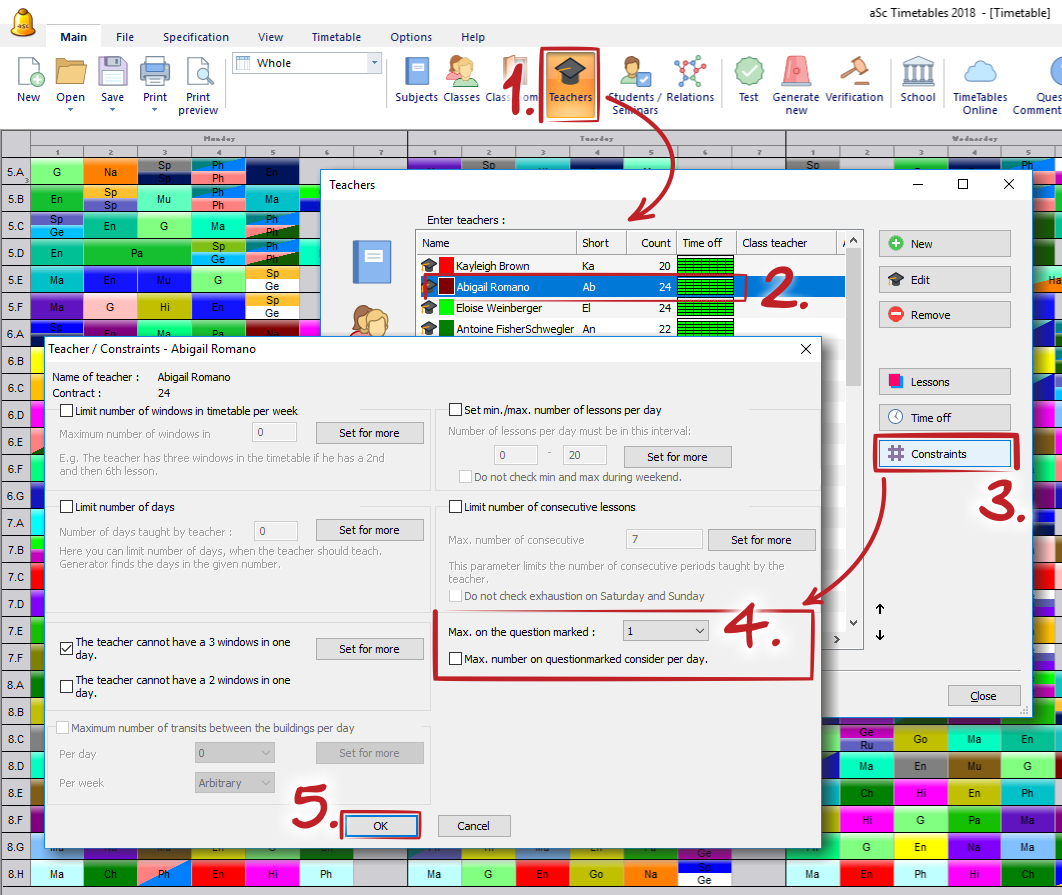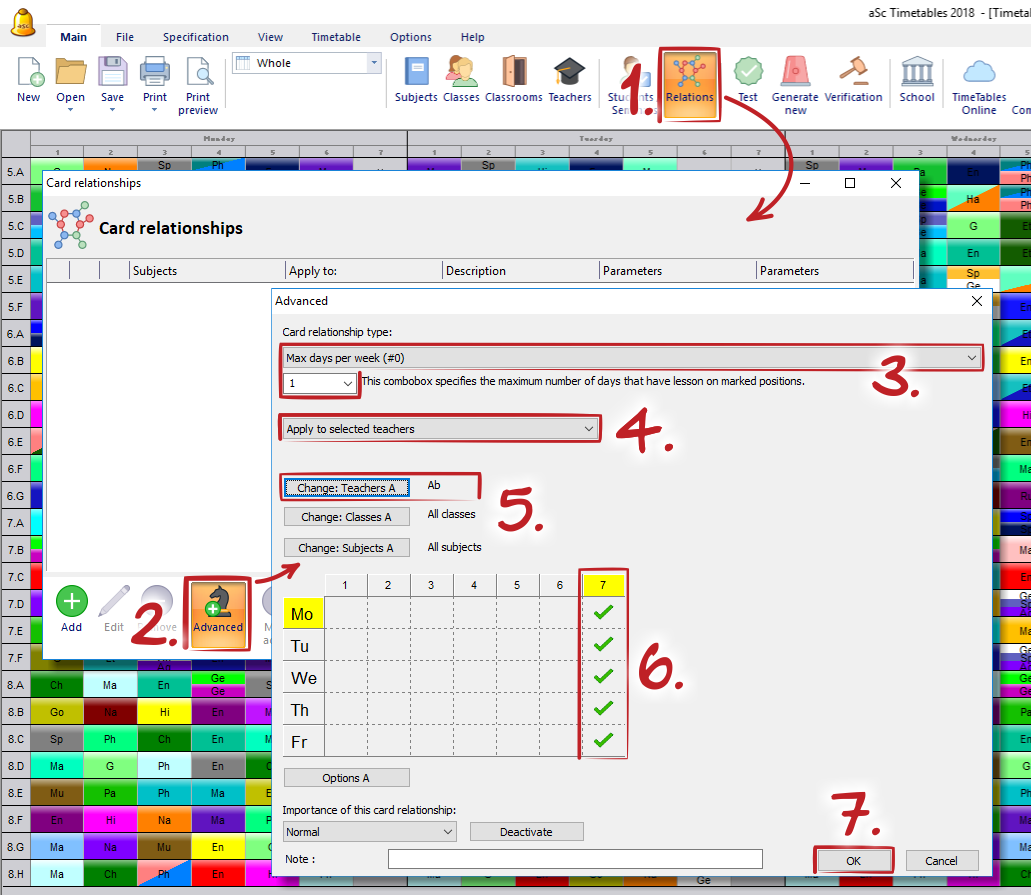#### aSc الجداول المدرسية - صفحة المساعدةaSc EduPage - Online HelpaSc Video HelpaSc الجداول المدرسية - Constraints - Teachers

How to specify a teacher can teach only one 7th lesson
هذا المقال متوفر حسب اللغات التالية :

Simple solution
Define the question marked positions:then define max. on quesion marked positions in teacher's details:You can specify how many lessons can be placed on question marked positions. You can e.g. specify that the teacher have 6th lesson question marked and max is 2. Then the teacher will have only two 6th lessons per week.

Using the check box you can tell the algorithm to consider the question marked count per day. E.g. you can say lessons 5th, 6th and 7th are question marked and the teacher can have max two per each day. Then you can be sure teacher has at least one free lesson to have a lunch each day.

Complex solution
You can use this advanced card relationship:تمت مشاهدة هذا المقال 108723 مرة57607 / 108723المقال السابق الرجوع الى صفحة المساعدة لدى aSc المقال التالي